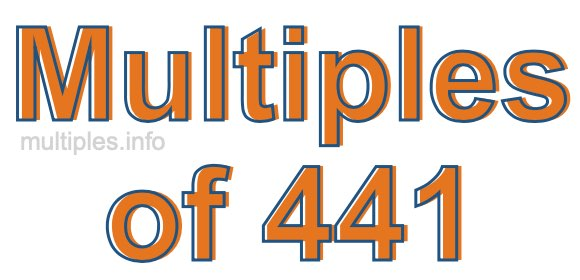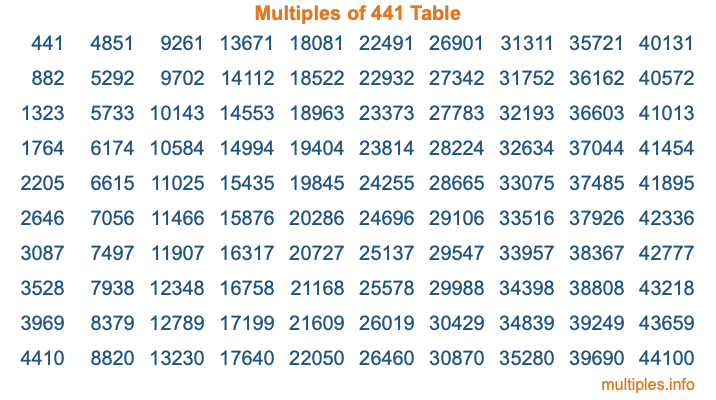Multiples of 441Welcome to the Multiples of 441 page. Here we will first teach you everything you will ever need to know about the multiples of 441, and then give you a study guide summary of everything we taught you to make sure you remember it all. Use this page to look up facts and learn information about the multiples of 441. This page will make you a multiples of four hundred forty-one expert!

Definition of Multiples of 441
Multiples of 441 are all the numbers that when divided by 441 equal an integer. Each of the multiples of 441 are called a multiple. A multiple of 441 is created by multiplying 441 by an integer.

Therefore, to create a list of multiples of 441, you start with 1 multiplied by 441, then 2 multiplied by 441, then 3 multiplied by 441, and so on for as long as you want. Thus, the list of the first five multiples of 441 is 441, 882, 1323, 1764, and 2205. To see a larger list of multiples of 441, see the printable image of Multiples of 441 further down on this page. We also have a category where you can choose any nth multiple of 441.

Multiples of 441 Checker
The Multiples of 441 Checker below checks to see if any number of your choice is a multiple of 441. In other words, it checks to see if there is any number (integer) that when multiplied by 441 will equal your number. To do that, we divide your number by 441. If the the quotient is an integer, then your number is a multiple of 441.

Is  a multiple of 441?

Least Common Multiple of 441 and ...
A Least Common Multiple (LCM) is the lowest multiple that two or more numbers have in common. This is also called the smallest common multiple or lowest common multiple and is useful to know when you are adding our subtracting fractions. Enter one or more numbers below (441 is already entered) to find the LCM.

Check out our LCM Calculator if you need more details about the Least Common Multiple or if you need the LCM for different numbers for adding and subtraction fractions.

nth Multiple of 441
As we stated above, 441 is the first multiple of 441, 882 is the second multiple of 441, 1323 is the third multiple of 441, and so on. Enter a number below to find the nth multiple of 441.

th multiple of 441

Multiples of 441 vs Factors of 441
441 is a multiple of 441 and a factor of 441, but that is where the similarities end. All postive multiples of 441 are 441 or greater than 441. All positive factors of 441 are 441 or less than 441.

Below is the beginning list of multiples of 441 and the factors of 441 so you can compare:

Multiples of 441: 441, 882, 1323, 1764, 2205, etc.

Factors of 441: 1, 3, 7, 9, 21, 49, 63, 147, 441

As you can see, the multiples of 441 are all the numbers that you can divide by 441 to get a whole number. The factors of 441, on the other hand, are all the whole numbers that you can multiply by another whole number to get 441.

It's also interesting to note that if a number (x) is a factor of 441, then 441 will also be a multiple of that number (x).

Multiples of 441 vs Divisors of 441
The divisors of 441 are all the integers that 441 can be divided by evenly. Below is a list of the divisors of 441.

Divisors of 441: 1, 3, 7, 9, 21, 49, 63, 147, 441

The interesting thing to note here is that if you take any multiple of 441 and divide it by a divisor of 441, you will see that the quotient is an integer.

Multiples of 441 Table
Below is an image of the first 100 multiples of 441 in a table. The table is in chronological order, column by column. The first column has the first ten multiples of 441, the second column has the next ten multiples of 441, and so on.The Multiples of 441 Table is also referred to as the 441 Times Table or Times Table of 441. You are welcome to print out our table for your studies.

Negative Multiples of 441
Although not often discussed or needed in math, it is worth mentioning that you can make a list of negative multiples of 441 by multiplying 441 by -1, then by -2, then by -3, and so on, to get the following list of negative multiples of 441:

-441, -882, -1323, -1764, -2205, etc.

Multiples of 441 Summary
Below is a summary of important Multiples of 441 facts that we have discussed on this page. To retain the knowledge on this page, we recommend that you read through the summary and explain to yourself or a study partner why they hold true.

There are an infinite number of multiples of 441.

A multiple of 441 divided by 441 will equal a whole number.

441 divided by a factor of 441 equals a divisor of 441.

The nth multiple of 441 is n times 441.

The largest factor of 441 is equal to the first positive multiple of 441.

441 is a multiple of every factor of 441.

441 is a multiple of 441.

A multiple of 441 divided by a divisor of 441 equals an integer.

441 divided by a divisor of 441 equals a factor of 441.

Any integer times 441 will equal a multiple of 441.

Multiples of a Number
Here you can get the multiples of another number, all with the same attention to detail as we did for multiples of 441 on this page.

Multiples of
Multiples of 442
Did you find our page about multiples of four hundred forty-one educational? Do you want more knowledge? Check out the multiples of the next number on our list!